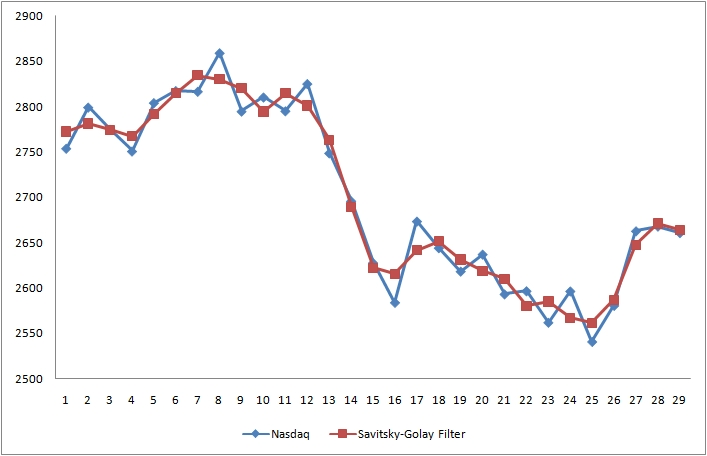# Savitsky-Golay Smoothing

This algorithm is a smoothing filter that essentially applies a polynomial regression of a certain degree to a time-series. The advantage of the Savitsky-Golay filter is that it tends to preserve certain features of the time-series like local minima and maxima.

The smoothing is computed using the algorithm described by Savitsky and Golay (A. Savitzky and Marcel J.E. Golay 1964. Smoothing and Differentiation of Data by Simplified Least Squares Procedures). The algorithm computes a local polynomial regression on the input data by solving the equation:

The method implemented in IPredict computes the Savitsky-Golay coefficients and then convolves them with the original time-series.

This is an example of the filter applied to the Nasdaq index:References:

A. Savitzky and Marcel J.E. Golay (1964). Smoothing and Differentiation of Data by Simplified Least Squares Procedures

 Forecasting Methods Holt Winter’s, Series Decomposition and Wavelet Benchmarks Time Series Forecasting Use of the Moving Average in Time-series Forecasting Forecasting Concepts Denoising Techniques Error Statistics Computational Performance Fast Fourier Transform Moving Averages Kernel Smoothing Active Moving Average Savitsky-Golay Smoothing Fractal Projection Downloading Financial Data from Yahoo Multiple Regression Digital Signal Processing Principal Component Analysis Curve Analysis Options Pricing with Black-Scholes Markowitz Optimal Portfolio Time-series preprocessing